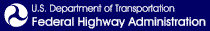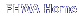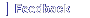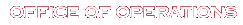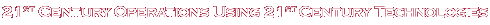# Calibration in Quantitative Alternatives Analysis

Printable version [PDF 6.0 MB]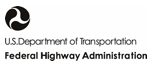U.S. Department of Transportation
Office of Operations
1200 New Jersey Avenue, SE
Washington, DC 20590
ops.fhwa.dot.gov

November 2020

FHWA-HOP-20-057

EXECUTIVE SUMMARY
CHAPTER 1. INTRODUCTIONBACKGROUNDOBJECTIVECHALLENGES AND SOLUTIONSCALIBRATION NEEDS
CHAPTER 2. REVIEW OF CALIBRATION METHODOLOGIESSCENARIO-BASED CALIBRATIONCORRELATED PARAMETERSTRAJECTORY-BASED CALIBRATIONCONCLUSIONS
CHAPTER 3. DATA ASSESSMENTDATA COLLECTION TECHNIQUESDATA PROCESSING AND ENHANCEMENTROLE OF VEHICLE TRAJECTORIES IN THE TAT CALIBRATION PROCEDURELOCAL DENSITY THROUGH VEHICLE TRAJECTORIESDATA REQUIREMENTSDATA STRUCTURECONCLUSIONS
CHAPTER 4. CALIBRATION NEEDSGAP ANALYSIS AND GAP IMPACTSIMPACTS OF THE GAPSCALIBRATION NEEDSCALIBRATION NEEDS LIBRARIESCONCLUSIONS
CHAPTER 5. METHODOLOGY AND FRAMEWORKOVERALL FRAMEWORKLIBRARY OF PARAMETERSAGENT AND SCENARIO GENERATION PROCESSSCENARIO-BASED ANALYSISROBUSTNESS-BASED ANALYSISCONCLUSIONS
CHAPTER 6. CASE STUDYACCELERATION (CAR FOLLOWING) FRAMEWORKCASE STUDY SCENARIOS AND AGENTSCONCLUSIONS
CHAPTER 7. STEP-BY-STEP APPROACH
CHAPTER 8. CONCLUSION
APPENDIX A. SUGGESTED NUMBER OF SCENARIOS AND NUMBER OF RUNS
APPENDIX B. USING BAYESIAN INFERENCE TO UPDATE SCENARIO PROBABILITIES
REFERENCES

### List of Figures

Figure 1. Formula. Relationship between inputs, outputs, and model parameters Formula. Fitness functions used as the objective function in calibration Formula. Weather effect coefficient in DYNASMART-P Diagram. Schematic representation of different conditions that impact a transportation network Formula. State-space formulation for generic online calibration Formula. Relationship between time mean speed and space mean speed Flowchart. The traffic analysis tools calibration procedure Formula. Relationship between density and space mean speed Formula. Space mean speed in the two-fluid theory Formula. Relationship between road density and fraction of stopped vehicles Formula. Density as a function of velocity and acceleration Illustration. Parameters with the least level of uncertainty [type 1] Illustration. Parameters with some level of uncertainty [type 2] Formula. Regret formulation Illustration. Parameters with the deep uncertainty [type 3] Flowchart. Overall framework Illustration. Process of developing a scenario/agent Flowchart. Proposed calibration framework Illustration. Constructing model output (travel time) distribution based on scenario-specific simulation outputs Formula. Newell car-following model (trajectory translation model) Formula. Gipps car-following model Formula. Helly car-following model Formula. The Intelligent Driver Model Formula. The stimulus-response acceleration model in MITSIMLab Formula. The Lane-Changing Model with Relaxation and Synchronization Formula. A probabilistic model for lane changing Formula. A gap acceptance model based on standard cumulative normal distribution Formula. A probit gap acceptance model for bicyclists and motorists Formula. Queue discharge rate in DYNASMART-P Graph. Type 1 modified Greenshields model (dual-regime model) Formula. Type 1 modified Greenshields model Graph. Type 2 modified Greenshields model (single-regime model) Formula. Type 2 modified Greenshields model Formula. Greenberg’s logarithmic model Formula. Underwood’s exponential model Formula. Pipes’ generalized model Formula. Weather effect adjustment of model parameters in DYNASMART Formula. Weather adjustment factor as a function of weather condition Formula. Scheduling cost in a demand model proposed by Frei et al. (2014) Formula. Link-level cost function proposed by Perez et al. (2012) Formula. A generalized mode choice utility function Formula. A time-of-day choice utility function Formula. A destination choice utility function Formula. Highway utility function proposed by Vovsha et al. (2013) Flowchart. Entity relationship diagram of the model parameter libraries Illustration. Process of generating agents and scenarios Flowchart. Scenario-based analysis Flowchart. Robustness-based analysis Illustration. I-290E study segment in Chicago, IL Formula. Value function for the uncongested regime Formula. Value function for the congested regime Formula. Binary probabilistic regime selection model Formula. Total utility function for the choice of acceleration Formula. Probability density function for the evaluation of drivers’ stochastic response Formula. The intelligent driver acceleration model Illustration. Radar sensor formation on an automated vehicle Formula. Maximum speed of automated vehicles Formula. Acceleration model for automated vehicles Diagram. Maximum safe speed curve Formula. Safe following distance formula Formula. Acceleration of automated vehicles Chart. Extended form of the lane-changing game with inactive vehicle-to-vehicle communication Diagrams. Compound figure depicts fundamental diagrams for different demand levels Equation. Weighted average travel time of the scenarios Charts. Compound figure depicts travel time distribution for mainline vehicles under different interarrival time scenarios Charts. Compound figure depicts fundamental diagrams for different levels of aggressiveness in driving behavior Charts. Compound figure depicts travel time distributions for mainline vehicles under different aggressive driving scenarios Diagram. Fundamental diagrams for different levels of aggressiveness in driving behavior Charts. Compound figure depicts travel time distribution for the mainline vehicles under various aggressive driver and conservative driver mix scenarios Charts. Compound figure depicts fundamental diagrams for a scenario in which the connected vehicle market penetration rate is 0 percent and the automated vehicle market penetration rate is 0 percent Charts. Compound figure depicts fundamental diagrams for a scenario in which the connected vehicle market penetration rate is 0 percent and the automated vehicle market penetration rate is 25 percent Charts. Compound figure depicts fundamental diagrams for a scenario in which the connected vehicle market penetration rate is 0 percent and the automated vehicle market penetration rate is 50 percent Charts. Compound figure depicts fundamental diagrams for a scenario in which the connected vehicle market penetration rate is 0 percent and the automated vehicle market penetration rate is 75 percent Charts. Compound figure depicts fundamental diagrams for a scenario in which the connected vehicle market penetration rate is 0 percent and the automated vehicle market penetration rate is 100 percent Charts. Compound figure depicts fundamental diagrams for a scenario in which the connected vehicle market penetration rate is 25 percent and the automated vehicle market penetration rate is 0 percent Charts. Compound figure depicts fundamental diagrams for a scenario in which the connected vehicle market penetration rate is 25 percent and the automated vehicle market penetration rate is 25 percent Charts. Compound figure depicts fundamental diagrams for a scenario in which the connected vehicle market penetration rate is 25 percent and the automated vehicle market penetration rate is 50 percent Charts. Compound figure depicts fundamental diagrams for a scenario in which the connected vehicle market penetration rate is 25 percent and the automated vehicle market penetration rate is 75 percent Charts. Compound figure depicts fundamental diagrams for a scenario in which the connected vehicle market penetration rate is 50 percent and the automated vehicle market penetration rate is 0 percent Charts. Compound figure depicts fundamental diagrams for a scenario in which the connected vehicle market penetration rate is 50 percent and the automated vehicle market penetration rate is 25 percent Charts. Compound figure depicts fundamental diagrams for a scenario in which the connected vehicle market penetration rate is 50 percent and the automated vehicle market penetration rate is 50 percent Charts. Compound figure depicts fundamental diagrams for a scenario in which the connected vehicle market penetration rate is 75 percent and the automated vehicle market penetration rate is 0 percent Charts. Compound figure depicts fundamental diagrams for a scenario in which the connected vehicle market penetration rate is 75 percent and the automated vehicle market penetration rate is 25 percent Charts. Compound figure depicts fundamental diagrams for a scenario in which the connected vehicle market penetration rate is 100 percent and the automated vehicle market penetration rate is 0 percent Equation. Travel time based on the Hurwicz optimism pessimism rule Diagram. Fundamental diagrams of mixed traffic scenarios Diagrams. Compound figure depicts average travel time at different market penetration rates for autonomous vehicles and connected vehicles on a selected segment of I-290 Diagram. Example for calculating the regret summation for the speed in the speed-density profile Diagram. Regret-based robustness metrics for different performance measures Diagram. Scenario rankings for various regret-based robustness metrics and performance measures Formula. Multivariate kernel density estimation formula Illustration. Compound figure depicts the process of smoothing the simulation output using the multivariate kernel density estimation method Formula. Relative mean integrated square error Formula. Minimum number of simulation runs for each scenario Formula. Bayes’ rule Formula. Relationship between prior and posterior states of mutually exclusive scenarios Formula. Revised Bayes’ rule Equation. Relationship between prior and posterior probabilities in example 1 Equation. Relationship between prior and posterior probabilities in example 2

## List of Tables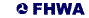United States Department of Transportation - Federal Highway Administration